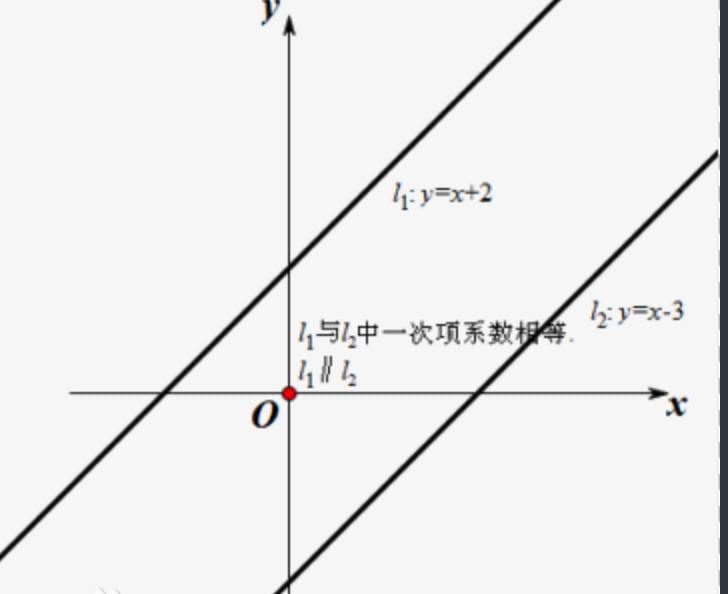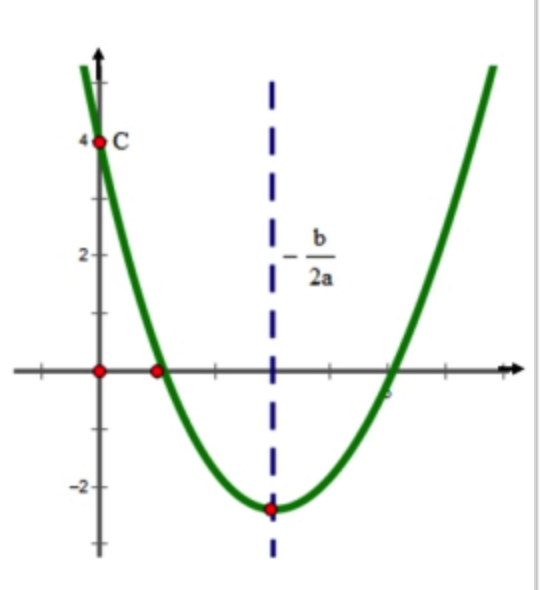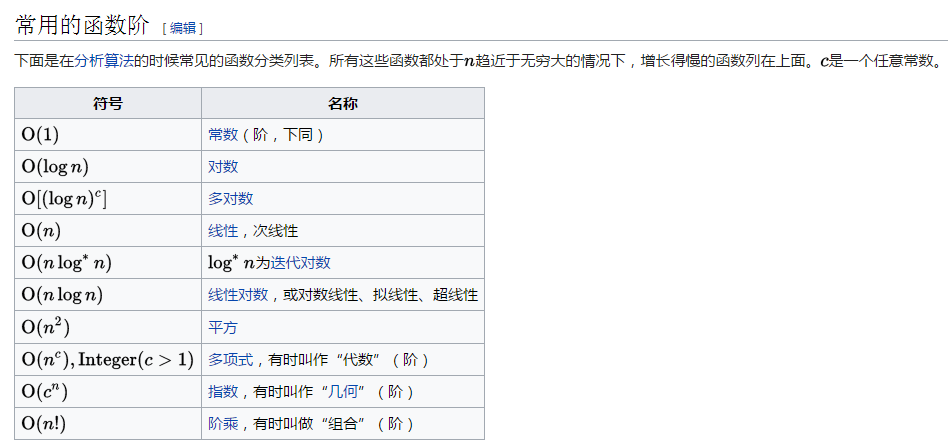# 时间复杂度：O(1)、O(n)、O(n²)、O(nlogn)等是什么意思，白话文解释专业术语。

（1）时间频度

10 这个值是固定的，就像去买菜，菜的单价是固定的，多少钱一斤。变化的值是你要买多少斤。

（2）时间复杂度

T(n) = n * X +5; （X 是执行一次循环体需要的时间，加个5可能是在执行for循环之前需要花费5的时间去做点初始化的事情。）

T(n)  /  f(n)  =  (n * 6 )   /   n   =   6

y = ax + b；T(n)  =  n * X +5;

T(n)  =  4n²  -  2n  + 2

T(n)  = O(f(n)) = O(n²)，

T(n) = n² * X + 10;  执行一次循环体需要X秒，再给10秒的时间初始化一下准备工作。

T(n)  = O(f(n)) = O(n²)

y =ax² + bx +cO(1)，说来也简单，也就是说这个的执行时间是不随着次数的增加而增加的，一般比如好多的单利模式，都只是初始化一次，然后一直的被使用。应该算是O(1)的例子吧。06-0734万+
03-09189407-0434万+
06-073676
07-0112万+
12-178万+
01-173503
09-206万+
05-177万+
05-292万+